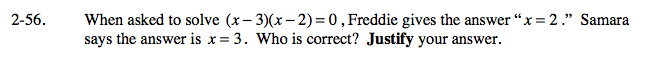Home > A2C > Chapter 2 > Lesson 2.1.4 > Problem2-56

2-56.

When asked to solve (x − 3)(x − 2) = 0 , Freddie gives the answer “x = 2.” Samara says the answer is x = 3. Who is correct? Justify your answer. Homework Help ✎Check both of their solutions in the equation.

They are both partially correct. The answer is x = 2 or x = 3.